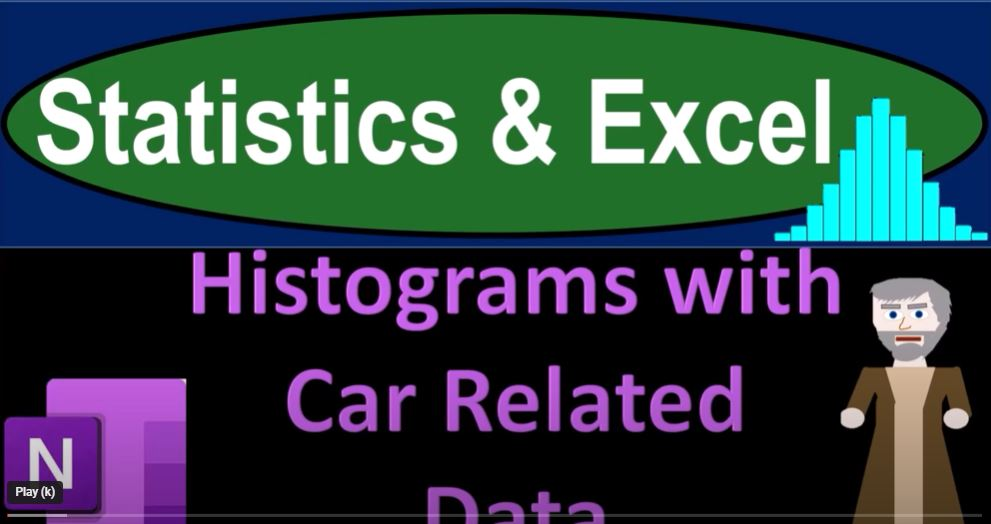## Histograms with Car Related Data 1056 Statistics & Excel

In the world of data analysis, extracting meaningful insights from raw data is a fundamental task. One powerful way to achieve this is by using statistical analysis and visualizations, such as histograms, to represent data. In this blog, we’ll dive into the fascinating world of car-related data and learn how to apply statistics and create Excel histograms to gain insights into this data. We’ll walk through various scenarios using different car-related attributes, exploring the average, median, maximum, and minimum values while creating histograms to visually represent the data distribution.

Sorting and Understanding Data

When dealing with datasets, the first step is often to sort the data in a meaningful way. For instance, with a dataset containing vehicle names and miles per gallon, sorting by miles per gallon helps organize the data and provides an initial glimpse. However, merely sorting may not provide sufficient insights. Therefore, we turn to statistics to gain a deeper understanding.

Calculating Average and Median

Using Excel, calculating the average (mean) and median of the miles per gallon values allows us to understand the central tendency of the data. The average is obtained by summing up all values and dividing by the count, while the median represents the middle value. These statistics offer insights into where the data is centered and how it’s distributed around that center.

Creating Histograms for Data Distribution

Histograms are an excellent tool to visualize data distribution. They divide data into ranges, called “buckets,” and display the frequency of values within each bucket. Excel’s histogram feature makes creating these visualizations a breeze. By simply selecting the dataset and choosing the histogram option, you can quickly generate a graphical representation of data distribution. This helps us identify trends, outliers, and the shape of the distribution.

Histograms are especially helpful when dealing with skewed data distributions. For instance, if the histogram skews to the right, it indicates a tail of higher values. This could imply outliers or unusual data points that influence the distribution.

Analyzing Cylinder Counts in Cars

Moving on to a dataset containing car names and the number of cylinders, we explore the average, median, maximum, and minimum cylinder counts. Here, the data is less varied, with cylinder counts typically ranging from 1 to 8. The median often provides a more representative center point than the average, as outliers might distort the latter. The data’s nature influences our choice between a traditional histogram and a bar chart. The bar chart might be more suitable for this data, allowing us to create custom ranges and better visualize the distribution.

Exploring Horsepower Variability

Lastly, we investigate another dataset featuring car names and their horsepower values. This data demonstrates greater variability, making it challenging to create a histogram with defined ranges. The maximum and minimum values, as well as the average and median, highlight the data’s spread. The histogram reveals the distribution’s shape, helping us identify clusters and anomalies.

Conclusion

In the realm of data analysis, statistics and histograms serve as invaluable tools for extracting insights from datasets. Our exploration of car-related data showcases the power of these tools in understanding data distribution, identifying trends, and uncovering outliers. By applying these techniques and creating visual representations, we gain a deeper understanding of the underlying patterns in the data. Whether it’s sorting, calculating statistics, or generating histograms, these steps empower us to transform raw data into meaningful insights that inform decision-making and further exploration.